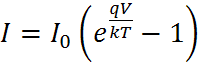# How to calculate solar cell efficiency with data in dark?

• nbky

#### nbky

Hi mates,
I have to find solar cell efficiency by datas that given. These datas comes from an experiment in light and in dark. I found efficiency in light by square method with IV curve.
But i don't know how to find in dark. The square method doesn't work cos data crossing the origin, no area to use this method.
I made some online research but haven't reached the right way.
Do you have any idea to calculate the efficiency?

I haven't find the solution for 5 hours.now i think there is no "efficiency in dark". I guess it behaves like diode and the "efficiency" is ideality factor. do you agree with me?
If it is so i have new problem:here the equation: i have voltage and current in my data. others are constant. And what is dark saturation current, i don't get it?

it s me again.i made some progress.
As you know the data is exponential, starts from origin (ectually close to origin). if i get the exponential equation from graph (like y=a*e^b), i could find I0 and "n".
So the n is ideality factor of solar cell. So do you think is it the answer?

I did my work and sent. And i get the feedback and i just want to inform you just in case maybe someone else stuck same kind of problem.

First of all i learned it was a tricky question. You cannot find efficiency just using data in dark. You need to combaine that with at least short circuit current under illumination. Then you have a theoretical model for IV model that you can calculate max power.

with only dark data you can only calculate saturation current and ideality factor which the question ask for from the begining. You can find it from the graph like i just said in the last post.